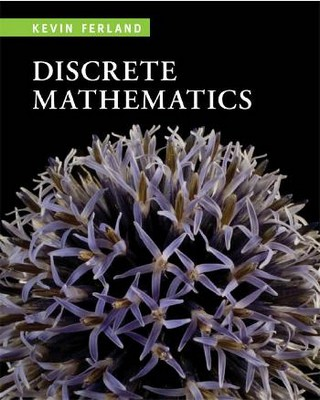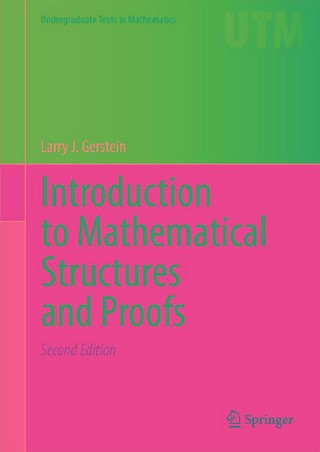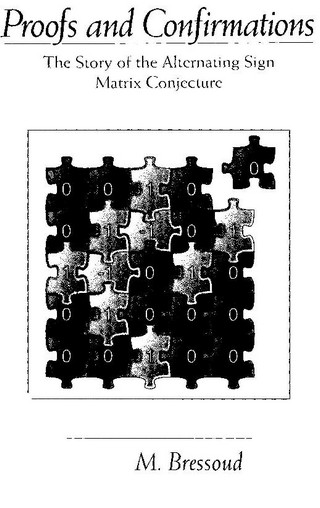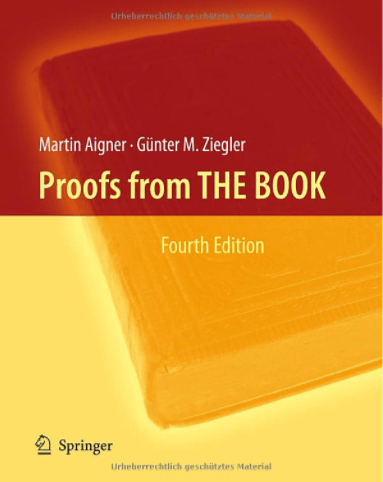Book Toankho

Sách hay - Sách đẹp

Browsing:

Tag: Proofs

An Introduction to Proofs and the Mathematical VernacularAn Introduction to Proofs and the Mathematical VernacularMartin V. Day Department of Mathematics Virginia Tech Blacksburg, Virginia 24061 http://www.math.vt.edu/people/day/ProofsBook/ Download ToanKho:http://lk.libvui.com/W9Ob http://megaurl.in/H84Bbo8

Discrete Mathematics (An Introduction to Proofs and Combinatorics)Discrete Mathematics: An Introduction to Proofs and Combinatorics by various. Houghton Mifflin College Div,2008 Download ToanKho:http://lk.libvui.com/5gC9lZ http://megaurl.in/rCig

Introduction to Mathematical Structures and ProofsAs a student moves from basic calculus courses into upper-division courses in linear and abstract algebra, real and complex analysis, number theory, topology, and so on, a “bridge” course can help ensure a smooth transition. Introduction to Mathematical Structures and Read more…

Algebra of Proofs (Studies in Logic and the Foundations of Mathematics)Here we study the algebraic properties of the proof theory of intuitionist first-order logic in a categorical setting. Our work is based on the confluence of ideas and techniques from proof theory, category theory, and combinatory logic, and this book Read more…

Proofs and Confirmations (The Story of the Alternating Sign Matrix Conjecture)This introduction to recent developments in algebraic combinatorics illustrates how research in mathematics actually progresses. The author recounts the dramatic search for and discovery of a proof of a counting formula conjectured in the late 1970s: the number of n Read more…

Proofs from THE BOOK (4th Edition)This revised and enlarged fourth edition of “Proofs from THE BOOK” features five new chapters, which treat classical results such as the “Fundamental Theorem of Algebra”, problems about tilings, but also quite recent proofs, for example of the Kneser conjecture Read more…